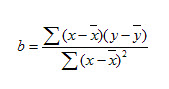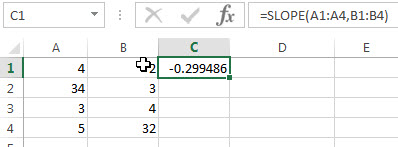# Excel SLOPE Function

This post will guide you how to use the SLOPE function with syntax and examples in Microsoft excel.

### Description

The Excel SLOPE function returns the slope of the linear regression line through data points in known_y’s and know_x’s. So you can use this function to calculate the slope of the linear regression line through a given set of x-values and y-values in Excel.

The SLOPE function is a build-in function in Microsoft Excel and it is categorized as a Statistical Function.

The SLOPE function is available in Excel 2016, Excel 2013, Excel 2010, Excel 2007, Excel 2003, Excel XP, Excel 2000, Excel 2011 for Mac.

### Syntax

The syntax of the SLOPE function is as below:

`= SLOPE (known_y's, known_x's)`

Where the SLOPE function arguments are:

• known_y’s -This is a required argument.  An array or cell reference that contain numeric known y-values used to calculate the slope.
• known_x’s -This is a required argument.  An array or cell reference that contain numeric known x-values used to calculate the slope.

Note:

• The argument must be numbers or arrays or cell references that contain only numbers.
• If any arguments contain text, logical values, or empty cells, those values will be ignored by the SLOPE function.
• If known_y’s and known_x’s arguments have a different lengths, the SLOPE function will return the #N/A Error.
• The equation for the slope of the regression line is:### Excel SLOPE Function Examples

The below examples will show you how to use Excel SLOPE Function to calculate the slope of the linear regression line through a given set of values.

1# to calculate the slope of the linear regression line through the data points in A1:A4 and B1:B4, using the following formula:

`=SLOPE (A1:A4,B1:B4)`